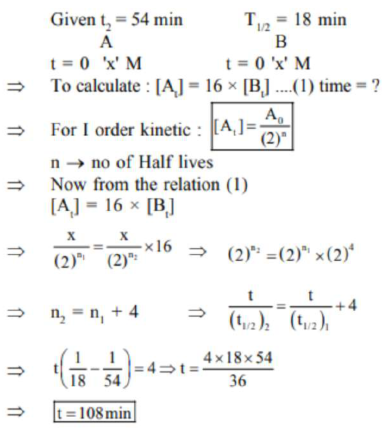# A and B decompose via first order kinetics with half-livesQuestion:

A and B decompose via first order kinetics with half-lives $54.0 \mathrm{~min}$ and $18.0 \mathrm{~min}$ respectively. Starting from an equimolar non reactive mixture of $\mathrm{A}$ and $\mathrm{B}$, the time taken for the concentration of $A$ to become 16 times that of $B$ is_____________ min. (Round off to the Nearest Integer).

Solution:

(108)